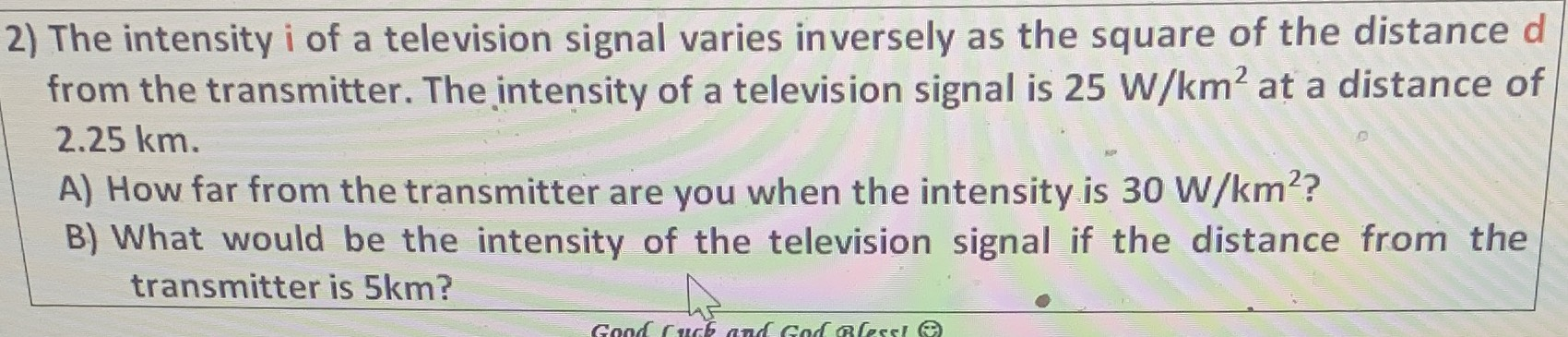### ¿Todavía tienes preguntas de matemáticas?

Pregunte a nuestros tutores expertos
Algebra
Pregunta2) The intensity i of a television signal varies inversely as the square of the distance d from the transmitter. The intensity of a television signal is $$25 W / km ^ { 2 }$$ at a distance of $$2.25 km$$ . A) How far from the transmitter are you when the intensity is $$30 W / km ^ { 2 }$$ ? B) What would be the intensity of the television signal if the distance from the transmitter is $$5 km$$ ?

$$i= \frac{k}{d} \Rightarrow k= id= 56.25\\i= 30\Rightarrow d= \frac{56.25}{30} = 1.875$$
$$d= 5\Rightarrow i= \frac{56.25}{5} = 11.25$$